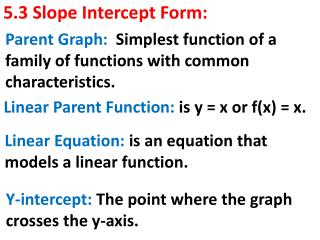DownloadDownload Presentation5.3 Slope Intercept Form:

# 5.3 Slope Intercept Form:

Download Presentation## 5.3 Slope Intercept Form:

- - - - - - - - - - - - - - - - - - - - - - - - - - - E N D - - - - - - - - - - - - - - - - - - - - - - - - - - -
##### Presentation Transcript

1. Parent Graph: Simplest function of a family of functions with common characteristics. 5.3 Slope Intercept Form: Linear Parent Function: is y = x or f(x) = x. Linear Equation: is an equation that models a linear function. Y-intercept: The point where the graph crosses the y-axis.

2. GOAL:

3. Whenever we are given a graph we must be able to provide the equation of the function. Slope-Intercept Form: The linear equation of a nonvertical line: y = m x + b Slope = = y-intercepty crossing

4. EX: Provide the equation of the line in slope-intercept form.?

5. Solution:The equation must be in slope-intercept form, that is in Y = mx + b B(3,8) 1. Find the y-intercept (b) A(0,5) In this graph b = + 5. 2. Find another point to get the slope. A(0,5) B(3,8)

6. Use the equation of slope to find the slope: = = = 1 B(3,8) The slope-intercept form equation is:y = 1x + 5 A(0,5) Remember:This means that if you start a 5 and move up one and over to the right one, and continue this pattern, you can get many points ON this line.

7. When work does not need to be shown: (EOC Test) look at the triangle made by the two points. B(3,8) A(0,5) Count the number of square going up or down and to the right. In this case 3up and 3 right. Thus slope is 3/3 = 1

8. YOU TRY IT:Provide the equation of the line in slope-intercept form.

9. YOU TRY IT: (Solution)The equation must be in slope-intercept form, that is in Y = mx + b A(0,4) 1. Find the y-intercept (b) In this graph b = + 4. 2. Find another point to get the slope. A(0,4) B(2,0) B(2,0)

10. Use the equation of slope to find the slope: = = = - 2 A(0,4) The slope-intercept form equation is:y = -2x + 4 B(2,0) Remember:This means that if you start a 4 and move down two and over to the right one, and continue this pattern, you can get many points ON this line.

11. When no work is required, you can use the rise/run of a right triangle between the two points: A(0,4) Look at the triangle, down 4 (-4) over to the right 2 (+2) slope = -4/+2 = -2 B(2,0) Remember:You MUST KNOW BOTH procedures, the slope formula and the triangle.

12. Given Two Points: We can also create an equation in the slope-intercept form from any two points: EX: Write the slope-intercept form of the line that passes through the points (3, -2) and(1, -3)

13. Use the given points and equation of slope: B(1,-3) A(3,-2) = = = - We now use the slope and a point to find the y intercept (b). y = mx + b -3 = - + b -3 += b Isolate b: b = - + = -

14. Going back to the equation: y = mx + b we replace what we have found: m = - and b = - To get the final slope-intercept form of the line passing through (3, -2) and(1, -3) y = -x -

15. We now proceed to graph the equation: y = -x - Y-intercepty crossing - 5 2

16. YOU TRY IT:Write the slope-intercept form of the line that passes through the points (-3, 4) and(2, -1)

17. Use the given points and equation of slope: B(1,-3) A(3,-2) = = = - We now use the slope and a point to find the y intercept (b). y = mx + b -3 = - + b -3 += b Isolate b: b = - + = -

18. Going back to the equation: y = mx + b we replace what we have found: m = - and b = - To get the final slope-intercept form of the line passing through (3, -2) and(1, -3) y = -x -

19. We now proceed to graph the equation: y = -x - Y-intercepty crossing - 5 2

20. CLASSWORK:Page 309-310 Problems: 2, 3, 6, 8, All ODDS .